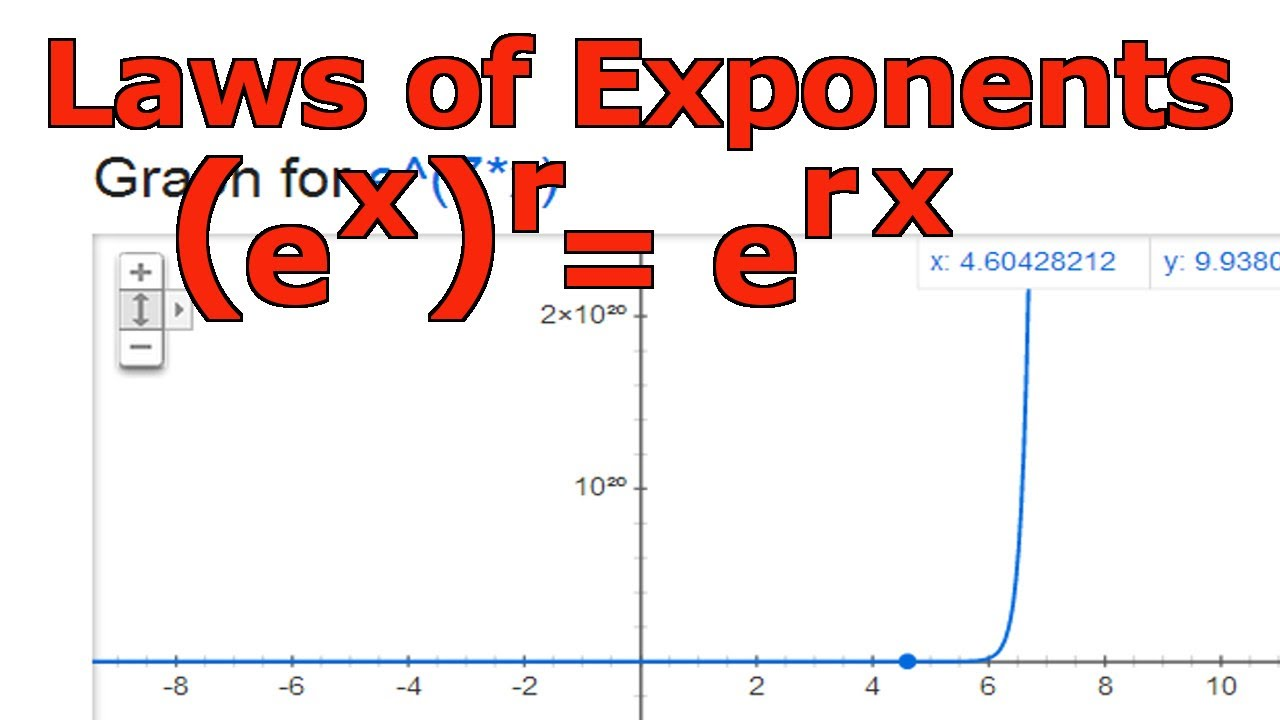# Laws of Exponents: (e^x)^r = e^(rx)In this video I go over another law of exponent and prove that (e^x)^r = e^(rx) where r is a rational number. In this proof I use a similar method as in my previous videos on the laws of exponents and utilize the natural logarithm function, ln(x), in simplifying and breaking down the function to obtain the exponent law.

View video notes on the Steem blockchain: https://steemit.com/hive-128780/@mes/laws-of-exponents-e-x-r-e-rx

Related Videos:

Laws of Exponents: e^(x+y) = e^x*e^y: http://youtu.be/8z4zpIjsVjk
Laws of Exponents: e^(x-y) = e^x/e^y: http://youtu.be/DpGjtkyQVPI
Laws of Logarithms: ln(x*y) = ln(x) + ln(y): http://youtu.be/2SCZzFy2b2s
Laws of Logarithms: ln(x/y) = ln(x) - ln(y): http://youtu.be/GXkyY9EmDGM
Laws of Logarithms: ln(x^r) = r*ln(x): http://youtu.be/mvJK8lQcdTY
Logarithms and their Properties - An Introduction: http://youtu.be/AZ6KKym19gI
Natural Logarithmns Defined as Integrals: Introduction: http://youtu.be/M-N2PQ5UZns
Inverse Functions Part 2: One to One Functions and the Horizontal Line Test: http://youtu.be/iSNuplUWrbA .

------------------------------------------------------

Become a MES Super Fan! https://www.youtube.com/channel/UCUUBq1GPBvvGNz7dpgO14Ow/join

DONATE! ʕ •ᴥ•ʔ https://mes.fm/donate

SUBSCRIBE via EMAIL: https://mes.fm/subscribe

MES Truth: https://mes.fm/truth
Official Website: https://MES.fm
Hive: https://peakd.com/@mes

Email me: [email protected]

Free Calculators: https://mes.fm/calculators

BMI Calculator: https://bmicalculator.mes.fm
Mortgage Calculator: https://mortgagecalculator.mes.fm
Percentage Calculator: https://percentagecalculator.mes.fm

Free Online Tools: https://mes.fm/tools

iPhone and Android Apps: https://mes.fm/mobile-apps